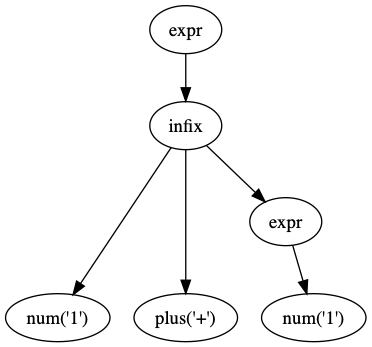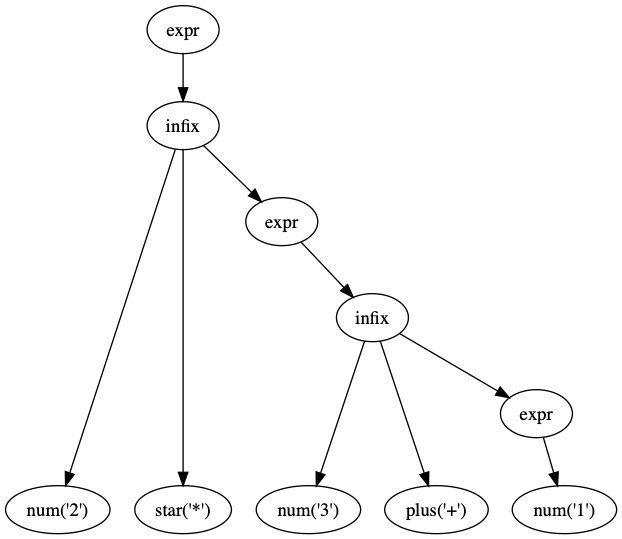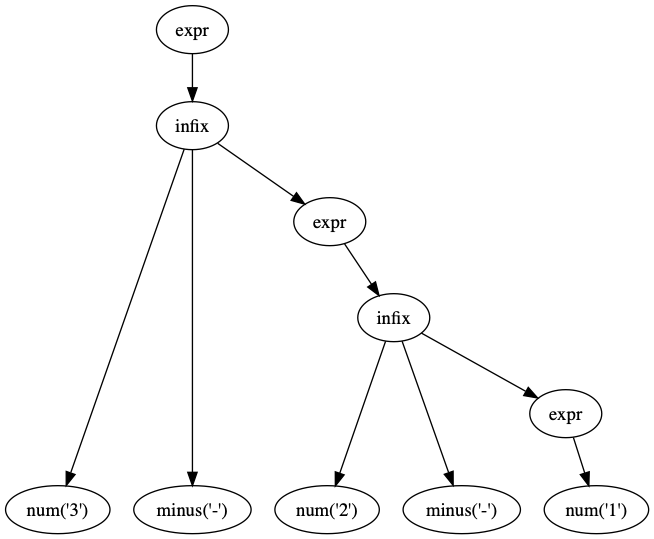Instantly share code, notes, and snippets.

Last active October 15, 2019 07:33
Messing around with lexing and parsing (mathterp.py)

Blog 2019/7/1

# Messing around with lexing and parsing (mathterp.py)

Revisiting this again with some co-workers.

This time around I've tried to split up the code into some reusable chunks.

## mathterp1.py

This grammar makes sense to us as humans, but demonstrates a weakness of recursive descent: that it can't do left recursion (it leads to infinite recursion).

``````# grammar:
#  expr:   infix | num
#  infix:  expr op expr
#  op:     plus | star
#  num:    /\d+/
#  plus:   /\+/
#  star:   /\*/
``````
``````\$ echo '1+1' | ./mathterp1.py
...
File "./mathterp1.py", line 48, in parse_infix
(ltree, tokens1) = parse_expr(tokens)
File "./mathterp1.py", line 61, in parse_expr
(tree, tokens1) = parse_infix(tokens)
File "./mathterp1.py", line 48, in parse_infix
(ltree, tokens1) = parse_expr(tokens)
File "./mathterp1.py", line 61, in parse_expr
(tree, tokens1) = parse_infix(tokens)
File "./mathterp1.py", line 48, in parse_infix
(ltree, tokens1) = parse_expr(tokens)
RuntimeError: maximum recursion depth exceeded
``````

The way to address this is by modifying the grammar. This brings up a trade-off with recursive descent: that you often end up creating an unintuitive grammar in order to satisfy the limitations of recursive descent.

## mathterp2.py

This grammar avoids the infinite loop because it is right-recursive.

``````# grammar:
#  expr:   infix | num
#  infix:  num op expr
#  op:     plus | star
#  num:    /\d+/
#  plus:   /\+/
#  star:   /\*/
``````
``````\$ echo '1+1' | ./mathterp2.py --graphviz
=> 2
``````However, it can't deal with operator precedence rules (`3+1` is evaluated first, which is incorrect):

``````\$ echo '2*3+1' | ./mathterp2.py --graphviz
=> 8
``````and it can't deal with associativity (`2-1` is evaluated first, which is incorrect):

``````\$ echo '3-2-1' | ./mathterp2.py --graphviz
=> 2
``````## mathterp3.py (TODO)

We can implement this grammar, which will address operator precedence, but still doesn't address associativity.

``````# grammar:
#  expr:    term
#  term:    factor plus term
#  factor:  num star factor
#  num:     /\d+/
#  plus:    /\+/
#  star:    /\*/
``````
This file contains bidirectional Unicode text that may be interpreted or compiled differently than what appears below. To review, open the file in an editor that reveals hidden Unicode characters. Learn more about bidirectional Unicode characters
 import sys def evaluate(tree): """evaluate the parse tree (calculate the answer).""" if tree is None: return None if "--ast" in sys.argv: import pprint pprint.pprint(tree) if "--dot" in sys.argv: print dot(tree), if "--graphviz" in sys.argv: run_graphviz(dot(tree)) def _eval(node): ntype = node["ntype"] children = node["children"] if ntype == "expr": return _eval(node["children"]) elif ntype == "num": return int(node["token"]["text"]) elif ntype == "plus": return lambda x,y: x + y elif ntype == "minus": return lambda x,y: x - y elif ntype == "star": return lambda x,y: x * y elif ntype == "slash": return lambda x,y: x / y elif ntype == "infix": l = _eval(children) op = _eval(children) r = _eval(children) return op(l,r) else: raise Exception("Don't know how to eval %s" % node) return _eval(tree) ### output def myprint(result): if result is not None: print "=>", result return result is not None def dot(tree): """represent the tree in graphviz "dot" notation.""" n =  # we stuff 'n' into an array to easily pass by reference leaves = [] def _dot(tree, i): root = "n%i" % n out = "" for child in tree["children"]: n += 1 nodenum = "n%i" % n if len(child["children"]): out += "\n %s [label=\"%s\"]" % (nodenum, child["ntype"]) else: out += "\n %s [label=\"%s\"]" % (nodenum, "%s(\'%s\')" % (child["ntype"], child["token"]["text"])) out += "\n %s -> %s;" % (root, nodenum) out += _dot(child, n) if len(tree["children"]) == 0: leaves.append(root) return out output = "// paste this into http://www.webgraphviz.com/" output += "\ndigraph parse_tree {" root = "n%i" % n output += "\n %s [label=\"%s\"]" % (root, tree["ntype"]) output += _dot(tree, n) output += "\n {rank = same; %s}" % " ".join(["%s;" % l for l in leaves]) output += "\n}\n" return output def run_graphviz(dot): with open("/tmp/ast.dot", "w") as f: f.write(dot) import subprocess subprocess.check_call("dot -Tpng /tmp/ast.dot > /tmp/ast.png", shell=True) subprocess.check_call("open /tmp/ast.png", shell=True)
This file contains bidirectional Unicode text that may be interpreted or compiled differently than what appears below. To review, open the file in an editor that reveals hidden Unicode characters. Learn more about bidirectional Unicode characters